# Statistics - Degree of freedom (df)

Generally, the degrees of freedom is always the number of terms that go into the formula minus one (The intercept).

The degrees of freedom is therefore the number of predictors that have a non-zero coefficients in the model.

Discover MoreR - Correlation

with: confidence interval df (degree of freedom) t-value p-value cor (Pearson's product-moment correlation)Statistics - (Student's) t-test (Mean Comparison)

The t-test is a test that compares means. NHST can be conducted yielding to a p-value Effect Size can be calculated like in multiple regression. Confidence Interval around the mean can also be...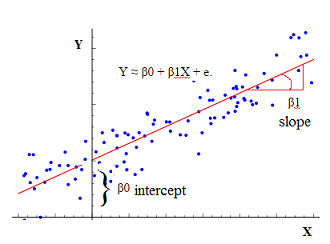Statistics - (Univariate|Simple|Basic) Linear Regression

A Simple Linear regression is a linear regression with only one predictor variable (X). Correlation demonstrates the relationship between two variables whereas a simple regression provides an equation...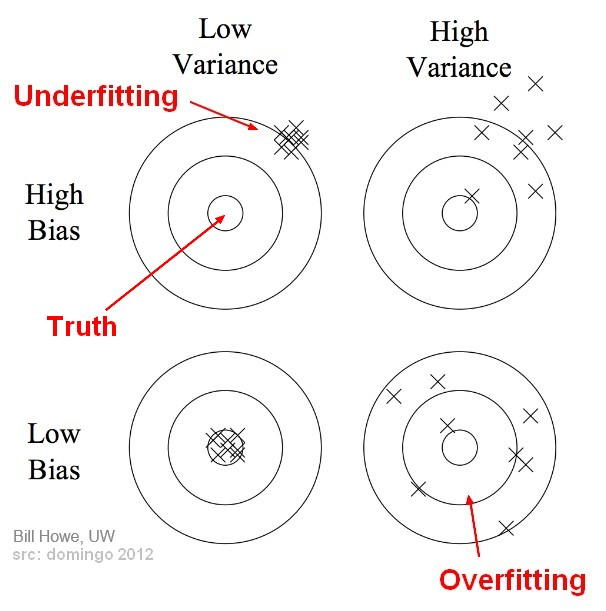Statistics - (Variance|Dispersion|Mean Square) (MS)

The variance shows how widespread the individuals are from the average. The variance is how much that the estimate varies around its average. It's a measure of consistency. A very large variance means...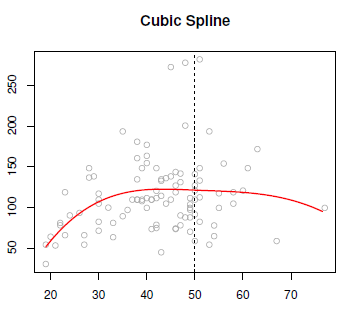Statistics - Cubic spline

Cubic splines is a step cubic piecewise polynomial in the left and the right and continuous at the knot. There's the following constraints: The first derivatives continues at the knot, and the...Statistics - Factorial Anova

A factorial ANOVA is done when the independent variables are categorical. By adding a second independent variable, we are entering in factorial ANOVA. N Independent Variables (IVs). Variables that...Statistics - Levene's test (Homogeneity variance test)

A Levene's test is essentially like a t-test but instead of comparing means it's comparing variances. If the test is significant, the homogeneity variance assumption is violated but we have a significant...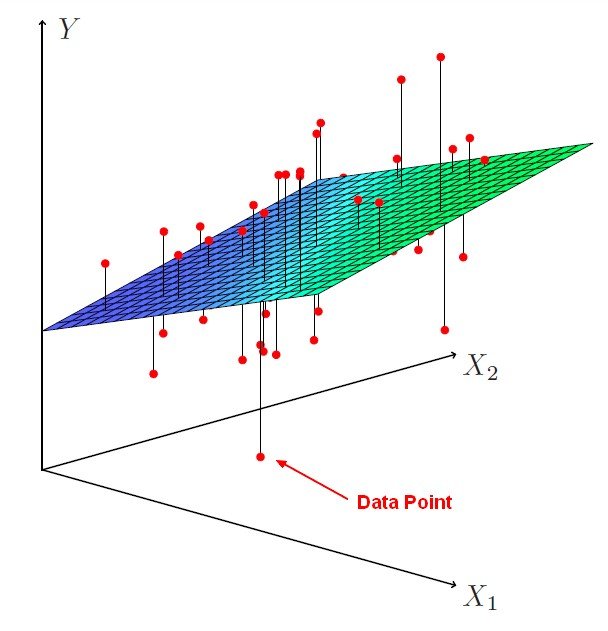Statistics - Multiple Linear Regression

Multiple regression is a regression with multiple predictors. It extends the simple model. You can have many predictor as you want. The power of multiple regression (with multiple predictor) is to better...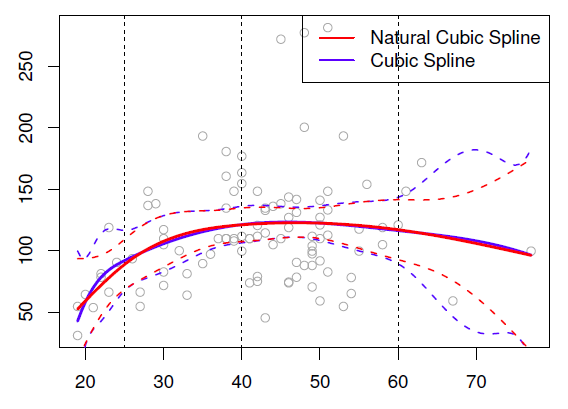Statistics - Natural (Cubic) Spline

A natural cubic spline is a cubic spline where two extra constraints have been added at the boundaries (on each end). The goal of this constraints is to avoid as for global cubic polynomial that the tail...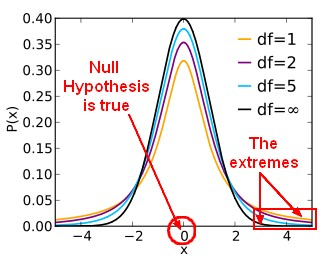Statistics - t-distributions

The t-distributions are used to calculate the p-value (basically you look it up in a table, nowadays software will compute it). It's basically a normal random variable except for small numbers of samples....